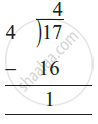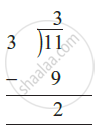# Types of Fraction - Improper Fraction and Mixed Fraction

• Improper Fraction
• Mixed Fraction
• Conversion between Improper and Mixed fraction

## Definition

Improper Fraction: The fractions, where the numerator is greater than the denominator are called improper fractions i.e., numerator > denominator. 3/2, 12/7, 18/5 are all examples of improper fractions.

Mixed Fraction: A mixed fraction has a combination of a whole and apart. 3 2/3, 4 1/4, 3 7/8 are all examples of mixed fractions.

## Notes

### Improper Fraction and Mixed Fraction:

#### 1. Improper Fraction:

• The fractions, where the numerator is greater than the denominator are called improper fractions i.e., numerator > denominator.
• Thus, fractions like 3/2, 12/7, 18/5 are all improper fractions.

#### 2. Mixed Fraction:

• A mixed fraction has a combination of a whole and apart.
• Thus, fractions like 3 2/3, 4 1/4, 3 7/8 are all mixed fractions.
• Mixed fraction will be written as "Quotient" ("Reminder"/"Divisor").

### i) Improper to mixed fraction:

1. 17/4 = (16 + 1)/4 = 16/4 + 1/4 = 4 + 1/4 = 4 1/4.i.e., 4 whole and 1/4  "more, or"  4 1/4.

2. 11/3 = (9 + 2)/3 = 9/3 + 2/3 = 3 2/3.i.e., 3 whole and 2/3  "more, or"  3 2/3.

3. 28/5 = (25 + 3)/5 = 25/5 + 3/5 = 5 + 3/5 = 5 3/5

4. 19/6 = (18 + 1)/6 = 18/6 + 1/6 = 3 + 1/6 = 3 1/6.

Thus, we can express an improper fraction as a mixed fraction by dividing the numerator by denominator to obtain the quotient and the remainder. Then the mixed fraction will be written as  "Quotient" ("Reminder"/"Divisor")

### ii) Mixed to Improper Fraction:

1. 10 3/5 = ((5 × 10) + 3)/5 = 53/5.

2. 9 3/7 = ((7 × 9) + 3)/7 = 66/7.

3. 8 4/9 = ((8 × 9) + 4)/9 = 76/9.

Thus, we can express a mixed fraction as an improper fraction as

(("Whole" × "Denominator") + "Numerator")/"Denominator".

or

"Quotient × Divisor + Reminder"/"Divisor".

If you would like to contribute notes or other learning material, please submit them using the button below.

### Shaalaa.com

Mixed Fraction [00:01:38]
S
0%